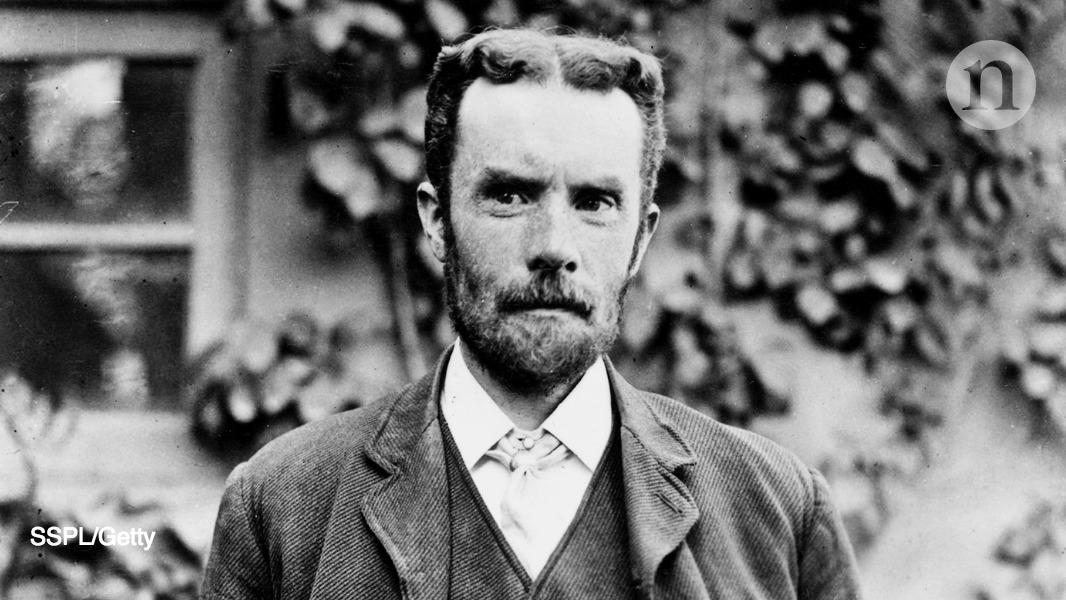# HeavisideOliver Heaviside (18 May 1850 - 3 February 1925) was a self-taught English electrical engineer, mathematician, and physicist who adapted complex numbers to the study of electrical circuits, invented mathematical techniques to the solution of differential equations (later found to be equivalent to Laplace transforms), reformulated Maxwell's field equations in terms of electric and magnetic forces and energy flux, and independently co-formulated vector analysis. Although at odds with the scientific establishment for most of his life, Heaviside changed the face of mathematics and science for years to come. Wikipedia, Heaviside

The term impedance was coined by Oliver Heaviside in July 1886.

ECE theory demonstrates that there are additional terms to be added to standard Maxwell Heaviside equations of conventional EM theory. These terms are the scalar and vector spin connection, ω0 and ω.
These terms can be considered as the time component of an axis of rotation and the space components of rotation respectively.
So, now the updated equation E = -(∇ + ω)Φ replaces the standard E = -∇Φ where Φ is the electric potential.
This added spin connection, ω allows electricity to be directly drawn from space as explained at the above mentioned websites.